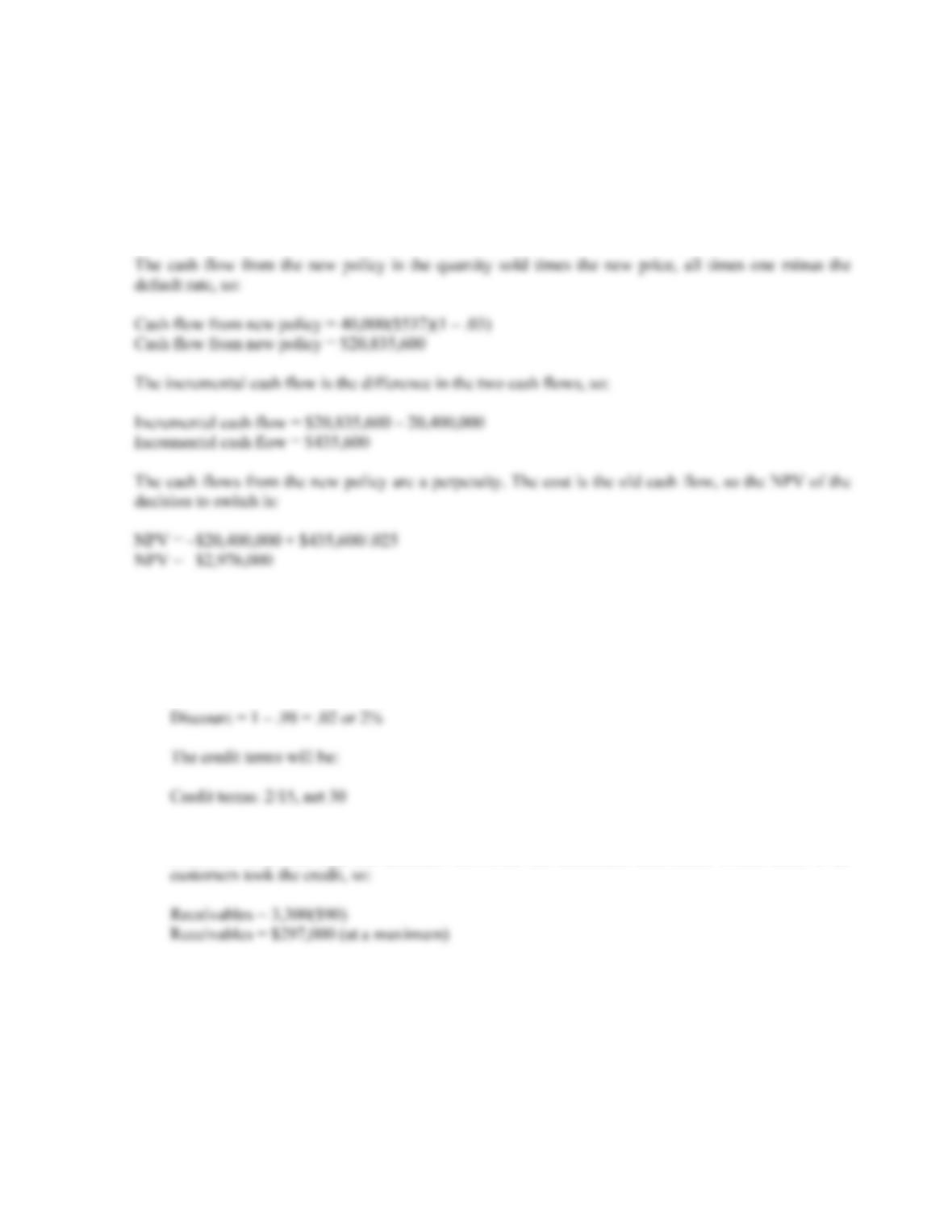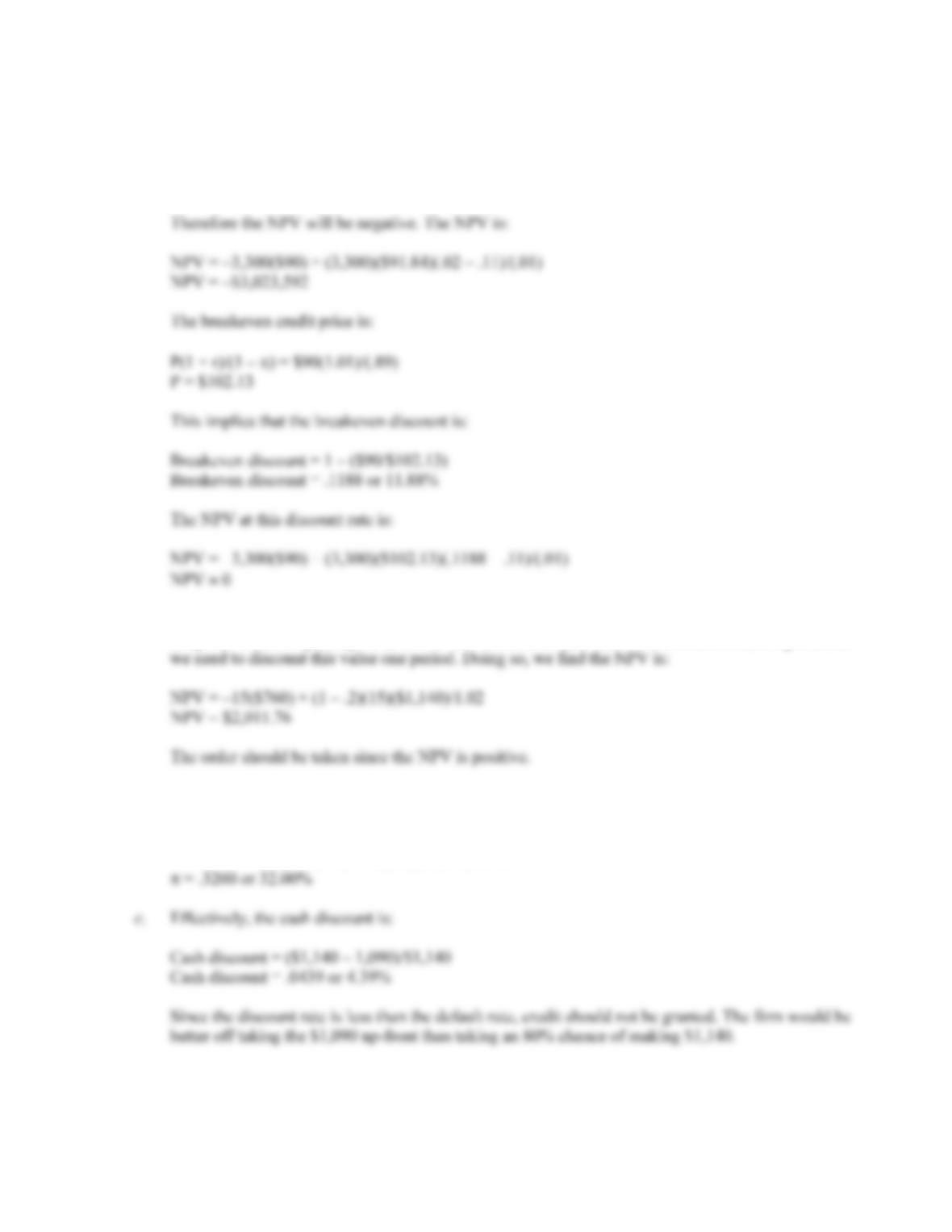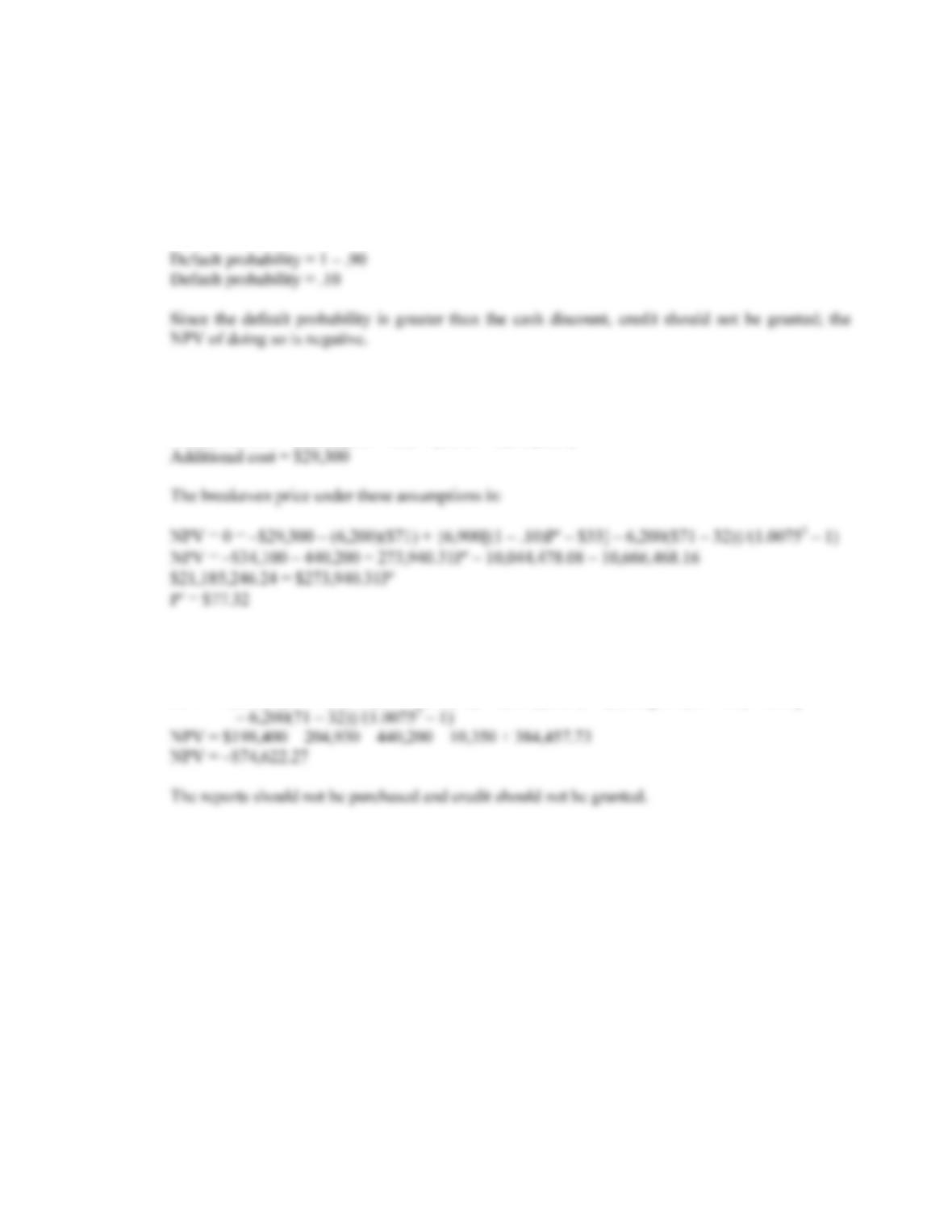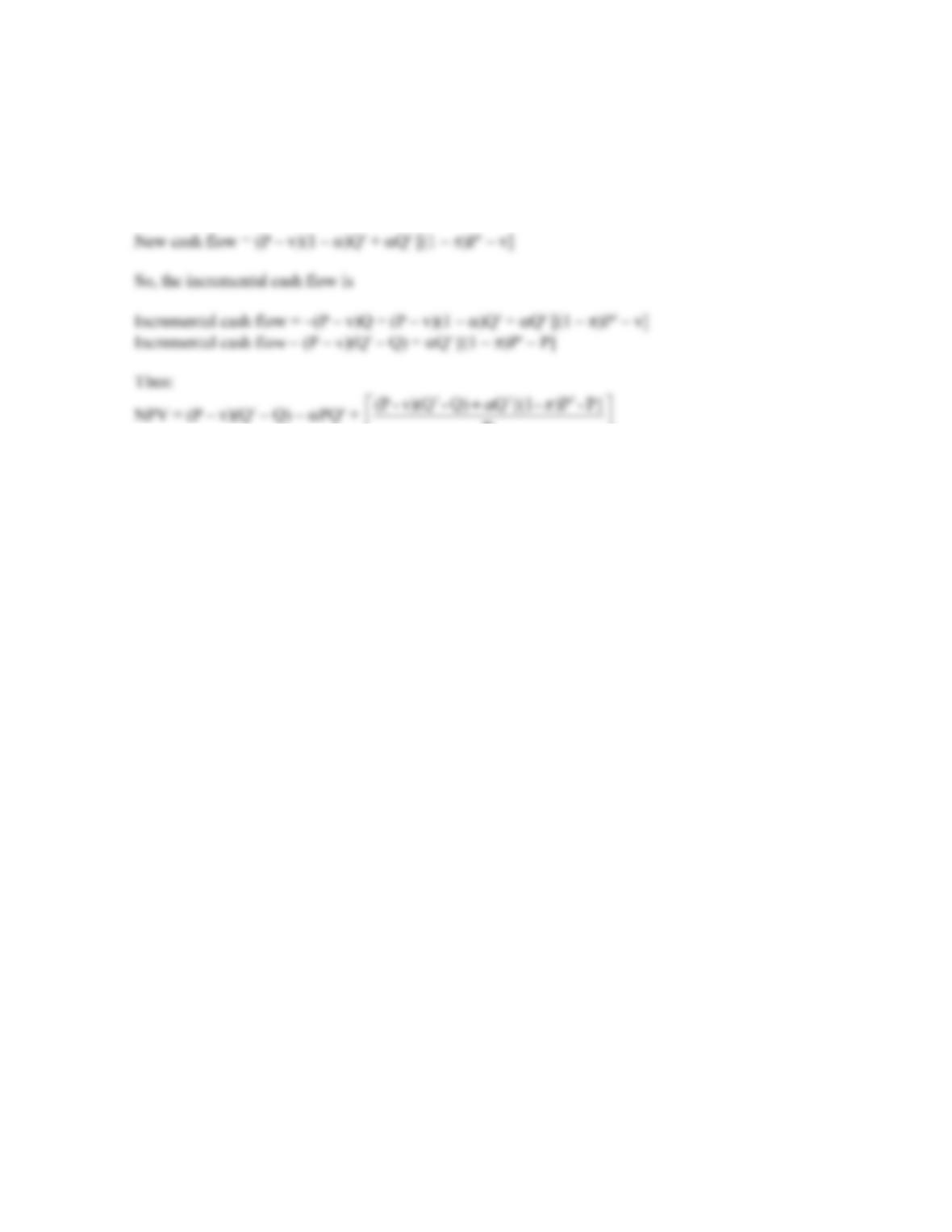Type
Quiz
Book Title
Fundamentals of Corporate Finance Standard Edition 9th Edition
ISBN 13
978-0073382395

### 978-0073382395 Chapter 20 Appendix

April 3, 2019B-340 SOLUTIONS
APPENDIX 20A
1. The cash flow from the old policy is the quantity sold times the price, so:
Cash flow from old policy = 40,000(\$510)
Cash flow from old policy = \$20,400,000
2. a. The old price as a percentage of the new price is:
\$90/\$91.84 = .98
So the discount is:
b. We are unable to determine for certain since no information is given concerning the percentage of
customers who will take the discount. However, the maximum receivables would occur if all
c. Since the quantity sold does not change, variable cost is the same under either plan.CHAPTER 20 B-341
d. No, because:
d
= .02 – .11
d
= –.09 or –9%
3. a. The cost of the credit policy switch is the quantity sold times the variable cost. The cash inflow is
the price times the quantity sold, times one minus the default rate. This is a one-time, lump sum, so
b. To find the breakeven default rate, , we just need to set the NPV equal to zero and solve for the
breakeven default rate. Doing so, we get:
NPV = 0 = –15(\$760) + (1 – )(12)(\$1,140)/1.02B-342 SOLUTIONS
4. a. The cash discount is:
Cash discount = (\$75 – 71)/\$75
Cash discount = .0533 or 5.33%
The default probability is one minus the probability of payment, or:
b. Due to the increase in both quantity sold and credit price when credit is granted, an additional
incremental cost is incurred of:
Additional cost = (6,200)(\$33 – 32) + (6,900 – 6,200)(\$33)
c. The credit report is an additional cost, so we have to include it in our analysis. The NPV when
using the credit reports is:
NPV = 6,200(32) – .90(6,900)33 – 6,200(71) – 6,900(\$1.50) + {6,900[0.90(75 – 33) – 1.50]CHAPTER 20 B-343
5. We can express the old cash flow as:
Old cash flow = (P – v)Q
And the new cash flow will be: